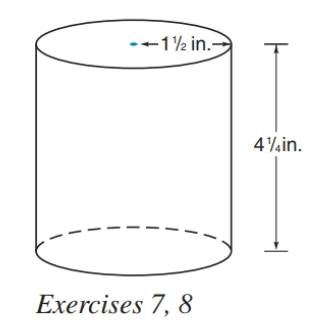Chapter 9.3, Problem 7EElementary Geometry For College St...

7th Edition
Alexander + 2 others
ISBN: 9781337614085

Solutions

Chapter
SectionElementary Geometry For College St...

7th Edition
Alexander + 2 others
ISBN: 9781337614085
Textbook Problem

The tin can shown at the right has the indicated dimensions. Estimate the number of square inches of tin required for its construction.(HINT: Include the lid and the base in the result.)To determine

To estimate:

The number of square inches of tin required for its construction.

Explanation

Given:

A tin can shown below has the indicated dimensions.

Formula used:

The total area of the right circular cylinder is T=2πrh+2πr2.

Calculation:

From the figure we see that, r=32in and h=174in.

Substitute the known values in the formula,

T=2πrh+2πr2=</

Still sussing out bartleby?

Check out a sample textbook solution.

See a sample solution

The Solution to Your Study Problems

Bartleby provides explanations to thousands of textbook problems written by our experts, many with advanced degrees!

Get Started

In Problems 17-24, find the slope and y-intercept and then graph each equation.

Mathematical Applications for the Management, Life, and Social Sciences

What is the defining characteristic of a repeated-measures or within-subjects research design?

Essentials of Statistics for The Behavioral Sciences (MindTap Course List)

Solve the equations in Exercises 126. 4xx313x4x31x31=0

Finite Mathematics and Applied Calculus (MindTap Course List)